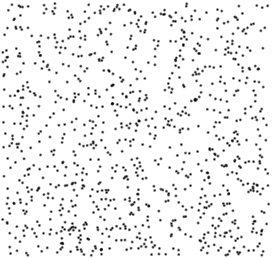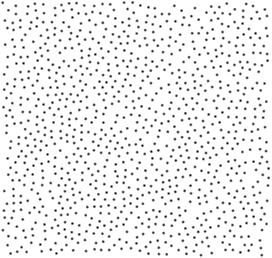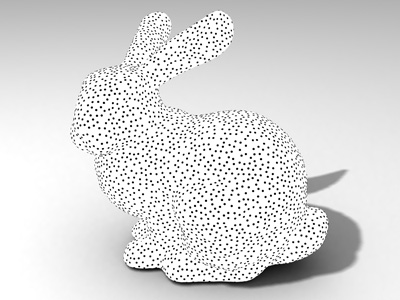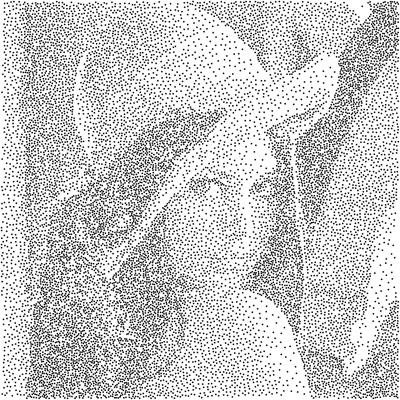# Poisson Disk Sampling

Poisson disk sampling has numerous uses in computer graphics and other fields. Here I will describe how to generate Poisson disk sample sets for any sampling problem using the cySampleElimination code release.

## What is Poisson Disk Sampling?

In a Poisson disk sample set no two samples are too close together. The closeness is defined by the Poisson disk radius, which is the half distance between the two closest samples. As compared to regular random sampling, Poisson disk sample sets provide a much more uniform sample distribution over the sampling domain.1000 Random Samples 1000 Poisson Disk Samples

The figure above shows a comparison of 1000 random samples to 1000 Poisson disk samples. Notice that Poisson disk sample points are more uniformly distributed over the sampling domain and no two sample points are too close together. Random samples, on the other hand, produce clusters and relatively large empty spaces between samples. This is not a deficiency of the particular random number generator used for this example. Uniform random samples provide uniform sample placement probability, but not a uniform coverage of the sampling domain.

Beyond providing a nice distribution of sample points, Poisson disk sample sets also provide superior convergence properties with Monte Carlo sampling. The same number of Poisson disk samples typically produces lower noise, as compared to random sampling.

## Generating Poisson Disk Sample Sets

The problem with Poisson disk sample sets is that generating them has been notoriously difficult. This is because samples cannot be generated independently; each sample depends on the positions of the other samples. Simple algorithms (like dart throwing) are computationally expensive. Computationally efficient algorithms are complex and limited to certain sampling domains. High-dimensional sampling was practically impossible for anything beyond 6 or 7 dimensions.

All of these problems of Poisson disk sample set generation has been resolved by the weighted sample elimination algorithm I introduced in 2015. You can find detailed information on this algorithm here: http://www.cemyuksel.com/research/sampleelimination/

In the remainder of this solution I will provide basic information about the weighted sample elimination algorithm and describe how the source code in the cySampleElimination code release can be used for generating Poisson disk sample sets for any sampling domain in any dimension.

## Weighted Sample Elimination

Weighted sample elimination is a simple algorithm for generating Poisson disk sample sets. One additional advantage of it is that it does not require specifying a Poisson disk radius property. Its input is a collection of (randomly generated) samples and it eliminates a portion of these samples such that the resulting sample set has Poisson disk property. In other words, the weighted sample elimination algorithm receives a large set of input samples and it returns a smaller set of output samples. It does not generate new samples. It merely selects a subset from a given input set.

The input samples can be generated for any sampling domain in any dimensions. The more input samples you provide, the better quality Poisson disk sample set you get, though beyond a certain percentage you get diminishing returns. I would recommend using an input set size no less than 3 times the output set size. I typically use 5 times the output set size, which provides a good balance between computation speed and sampling quality. That said, it is not a good idea to use a very small input sample set, regardless of the output sample set size. For example, if you would merely generate 10 samples, using 50 input samples may not provide a good input sample distribution. In this case, it would be a good idea to start with a much larger input sample set size.

## Using cySampleElimination

The cySampleElimination code release includes a template class that can work with any given sample class type, though it is designed to work with classes in the cyPoint code release (Point2 for 2D, Point3 for 3D, Point4 for 4D, and Point for arbitrary dimensions).

The first thing to do is to generate an input sample set. Let's say that we would like to have 1000 output samples, so we will begin with generating 5000 input samples. How you generate these samples is entirely up to you and your sampling problem. Below I include a simple code for generating random samples in 3D using Point3f.

```std::vector< cy::Point3f > inputPoints(5000);
for ( size_t i=0; i<inputPoints.size(); i++ ) {
inputPoints[i].x = (float) rand() / RAND_MAX;
inputPoints[i].y = (float) rand() / RAND_MAX;
inputPoints[i].z = (float) rand() / RAND_MAX;
}
```

Notice that all sample values are between zero and one. This is important. If your sampling domain is different, you will need to specify its dimensions. I will discuss this below. For now, let's assume that we would like all sample values to be between zero and one.

For using weighted sample elimination, I first need to create an instance of the WeightedSampleElimination class.

```cy::WeightedSampleElimination< Point3f, float, 3, int > wse;
```

The template parameters here define the sample class type (in this case `Point3f`), floating point type (`float` or `double`), the dimensionality of the point type (in this case 3), and the type for the sample index (in this case `int`). Unless you are working with more than 2 billion samples, sample index type `int` will suffice.

The constructor will set the default parameters. You can alter them if you like. I will talk about the parameters below. For now, let's assume that we are happy with the default parameters. For generating a Poisson disk sample set, all I need to do is to call the `Eliminate()` method of the WeightedSampleElimination class.

```std::vector< cy::Point3f > outputPoints(1000);
wse.Eliminate( inputPoints.data(), inputPoints.size(),
outputPoints.data(), outputPoints.size() );
```

That's it! The output points will have Poisson disk property. Notice that we didn't have to specify a Poisson disk radius. The eliminate method can take a few more parameters. I will discuss them below.

## Progressive sampling

For some sampling problems it is desirable to introduce samples one by one, and stop introducing new samples once a desired property is reached. This is called progressive sampling (or adaptive sampling). The `Eliminate()` method can easily produce a progressive sample set by setting its `progressive` argument as `true`.

```wse.Eliminate( inputPoints.data(), inputPoints.size(),
outputPoints.data(), outputPoints.size(),
true );
```

In this case the samples in the output set is ordered, such that when the samples are introduced one by one in this order, each subset in the sequence becomes a Poisson disk sample set. The animation below shows an example sequence.Progressive Samples

## Sampling Arbitrary Volumes

As indicated above, our example sample points had values between zero and one. If you would like to sample a different domain, you must specify the bounds of your domain. For example, let's assume that our sampling domain is a box in 3D, where the minimum bound is (-2,-1,0) and the maximum bound is (2,1,1). We can set these bounds, as shown below.

```cy::Point3f minimumBounds(-2,-1, 0);
cy::Point3f maximumBounds( 2, 1, 1);
wse.SetBoundsMin( minimumBounds );
wse.SetBoundsMax( maximumBounds );
```

Note that these bounds specify a box-shaped domain. If you would like to sample an arbitrary volume in 3D, setting these bounds won't help. Instead, we must directly specify the `d_max` argument of the `Eliminate()` method. The `d_max` argument determines the maximum possible distance between two samples in the sampling domain for the desired number of output samples. This is a well-defined value in 2D and 3D, and it can be approximated in higher dimensions. The `GetMaxPoissonDiskRadius()` method helps with this parameter. The `d_max` argument should be twice the radius value that this method returns.

```float d_max = 2 * wse.GetMaxPoissonDiskRadius( 3, outputPoints.size(), volume );
wse.Eliminate( inputPoints.data(), inputPoints.size(),
outputPoints.data(), outputPoints.size(),
isProgressive,
d_max );
```

The parameters of the `GetMaxPoissonDiskRadius()` method are the dimensions, the output sample size, and the volume of the sampling domain. If the volume is given as zero (the default argument value), the volume is computed using the bounds. Otherwise, the bounds are completely ignored.

## Sampling Surfaces in 3D

The last argument of the `Eliminate()` method can be used for sampling surfaces in 3D (or low-dimensional manifolds in a high-dimensional space). First of all, the input points must be generated on the desired surface. Then, while calling the `Eliminate()` method, we must specify both the correct `d_max` argument and the dimensionality of the sampling domain. In our example, since we are sampling a surface in 3D, the sampling domain should be considered 2D.

```float d_max = 2 * wse.GetMaxPoissonDiskRadius( 2, outputPoints.size(), area );
wse.Eliminate( inputPoints.data(), inputPoints.size(),
outputPoints.data(), outputPoints.size(),
isProgressive,
d_max, 2 );
```

In this case, the `area` variable holds the total surface area of the sampling domain.

Notice that the WeightedSampleElimination object was created as a 3D sampler (working on samples in 3D), but we specify the dimensionality of the sampling domain as 2D, since we would like to sample a surface. That said, if `d_max` is specified and progressive sampling is not used, the last argument that specifies the dimensionality argument is not used.Samples generated on a Stanford bunny model

## Tiling

It is sometimes desirable to generate a sample set and then tile it over a domain. Also, when using statistical analysis using power spectrum, the generated sample set must be tileable. Tiling involves some extra computation; therefore, it is off by default. You can turn tiling on using the `SetTiling()` method.

Note that if tiling is off, weighted sample elimination would produce more output sample sets near the boundaries of the sampling domain. Therefore, it is a good idea to turn on tiling for box-shaped sampling domains, even if the sampling problem does not involve tiling.

Sometimes it is desirable to have a different sampling density at different parts of a domain. We can achieve this by adjusting the weight function accordingly. I will not provide more details on this beyond saying that you can use a custom weight function. This custom weight function should return a larger value for the parts of the domain that should be sampled more sparsely and should return a smaller value for the parts of the domain with a denser desired sample distribution. You will also need to provide a custom `d_max` argument, which should be the value for the most sparsely sampled area.Samples generated using a density map

The default weight function uses three parameters: `alpha`, `beta`, and `gamma`. The `alpha` parameter is the exponent used for the weight function. Its default value is 8. You probably don't need to modify this parameter at all.
The `beta` parameter specifies the amount of weight limiting. Weight limiting is a method that can provide higher quality sample sets, but aggressive weight limiting can sometimes generate a few samples that are closely placed, which is often undesirable. By default, weight limiting is on and the `beta` parameter is set to 0.65. The value of the `beta` parameter should be between zero and one. Larger values provide more aggressive weight limiting and smaller values diminish the impact of weight limiting. Setting `beta` to zero is the same as turning off weight limiting.
Finally, the `gamma` parameter is used for determining the limit for weight limiting based on the relative ratio of input and output set sizes.